+

# Introduction to Absolute Value

##### Rating:
(1)
• (0)
• (1)
• (0)
• (0)
• (0)
Author: Sophia Tutorial
##### Description:

Determine the result of an expression with absolute value.

(more)
Tutorial
what's covered
1. Introduction to Absolute value
2. Adding and Subtracting with Absolute Value
3. Multiplying and Dividing with Absolute Value

# 1. Introduction to Absolute Value

The absolute value of a number is its distance from zero on the number line. To illustrate this, let's take a look at a number on the number line, and identify its distance from zero:As we can see in the number line above, the number 4 is 4 units away from zero. This means that the absolute value of 4 is 4. This seems easy, and with positive numbers, it is very straightforward. But what about -4? The magnitude of the number is the same (the actual number we see, ignoring if it's positive or negative), but it is on the opposite side of 0 on the number line, as shown below:We see that -4 is also 4 units away from 0 on the number line. This means that the absolute value of -4 is 4. Notice that with both 4 and -4, the absolute value is non-negative.

term to know
Absolute value
The distance between a number and zero on the number line; it is always non-negative.

big idea
When taking the absolute value of a number, it is always non-negative. A simple way to think about evaluating the absolute value of a number is to rewrite the number without regard to its sign (do not write positive or negative, just the number).

# 2. Adding and Subtracting with Absolute Value

Now that we have an understanding of the absolute value of a number, let's use absolute value in addition and subtraction problems. The important thing here is to evaluate the absolute value first, and then perform the addition or subtraction. This is shown in the following examples: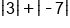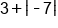Evaluate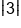as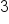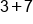Evaluate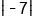as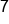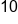Our Solution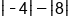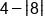Evaluate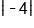as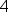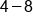Evaluate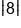as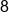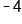Our Solution

If there are multiple number and operations within absolute value bars, we must evaluate the expression inside before taking any absolute value. This is because absolute value bars also act as grouping symbols, and must be evaluated first according to the order of operations.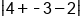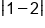Add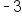to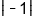Subtract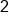from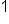Our Solution

# 3. Multiplying and Dividing with Absolute Value

There are a couple of special properties with absolute value when multiplying and dividing two numbers. Let's take a look at multiplication first: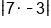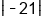Multiplyby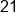Our Solution

Let's take a look at another way to approach the same problem: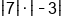Write as two absolute values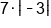Evaluate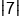as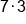Evaluate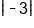asOur Solution

big idea
With products, we can say that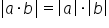.

Next, let's see if the same property holds true with division: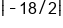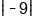Divide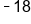by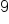Our Solution

Do you think we will arrive at the same solution if we first rewrite the expression using two sets of absolute value bars?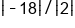Write as two absolute values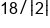Evaluate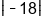as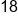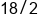Evaluate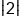asOur Solution

big idea
With quotients, we can say that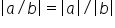.

summary
Absolute value is defined as the distance a number is away from 0 on a number line. Because it's a distance, it will always be a non-negative number. In other words, it will either be zero or a positive number. The absolute value sign acts as a grouping symbol. When you're adding and subtracting with absolute values, you would do things in the absolute value symbol first, just like parentheses. Finally, when multiplying and dividing absolute values, the product quotient properties of absolute value will allow us to simplify absolute value expressions.

Terms to Know
Absolute Value

The distance between a number and zero on the number line; it is always non-negative.

Rating Header